# RTransferEntropy

## Introduction to RTransferEntropy

The measurement of information transfer between different time series is the basis of research questions in various research areas, including biometrics, economics, ecological modelling, neuroscience, sociology, and thermodynamics. The quantification of information transfer commonly relies on measures that have been derived from subject-specific assumptions and restrictions concerning the underlying stochastic processes or theoretical models. With the development of transfer entropy, information theory based measures have become a popular alternative to quantify information flows within various disciplines. Transfer entropy is a non-parametric measure of directed, asymmetric information transfer between two processes.

We show how to quantify the information flow between two stationary time series and how to test for its statistical significance using Shannon transfer entropy and Rényi transfer entropy within the package RTransferEntropy. A core aspect of the provided package is to allow statistical inference and hypothesis testing in the context of transfer entropy. To this end, we first present the methodology, i.e. the derivation and calculation of transfer entropy as well as the associated bias correction applied to calculate effective transfer entropy, and describe our approach to statistical inference. Afterwards, we introduce the package in detail and demonstrate its functionality in several applications to simulated processes as well as an application to financial time series.

## Measuring information flows using transfer entropy

Let $$log$$ denote the logarithm to the base 2, then informational gain is measured in bits. Shannon entropy (Shannon 1948) states that for a discrete random variable $$J$$ with probability distribution $$p(j)$$, where $$j$$ stands for the different outcomes the random variable $$J$$ can take, the average number of bits required to optimally encode independent draws from the distribution of $$J$$ can be calculated as

$H_J = - \sum_j p(j) \cdot log \left(p(j)\right).$

Strictly speaking, Shannon’s formula is a measure for uncertainty, which increases with the number of bits needed to optimally encode a sequence of realizations of $$J$$. In order to measure the information flow between two processes, Shannon entropy is combined with the concept of the Kullback-Leibler distance (Kullback and Leibler 1951) and by assuming that the underlying processes evolve over time according to a Markov process (Schreiber 2000). Let $$I$$ and $$J$$ denote two discrete random variables with marginal probability distributions $$p(i)$$ and $$p(j)$$ and joint probability distribution $$p(i,j)$$, whose dynamical structures correspond to stationary Markov processes of order $$k$$ (process $$I$$) and $$l$$ (process $$J$$). The Markov property implies that the probability to observe $$I$$ at time $$t+1$$ in state $$i$$ conditional on the $$k$$ previous observations is $$p(i_{t+1}|i_t,...,i_{t-k+1})=p(i_{t+1}|i_t,...,i_{t-k})$$. The average number of bits needed to encode the observation in $$t+1$$ if the previous $$k$$ values are known is given by

$h_I(k)=- \sum_i p\left(i_{t+1}, i_t^{(k)}\right) \cdot log \left(p\left(i_{t+1}|i_t^{(k)}\right)\right),$ where $$i^{(k)}_t=(i_t,...,i_{t-k+1})$$. $$h_J(l)$$ can be derived analogously for process $$J$$. In the bivariate case, information flow from process $$J$$ to process $$I$$ is measured by quantifying the deviation from the generalized Markov property $$p(i_{t+1}| i_t^{(k)})=p(i_{t+1}| i_t^{(k)},j_t^{(l)})$$ relying on the Kullback-Leibler distance (Schreiber 2000). Thus, (Shannon) transfer entropy is given by

$T_{J \rightarrow I}(k,l) = \sum_{i,j} p\left(i_{t+1}, i_t^{(k)}, j_t^{(l)}\right) \cdot log \left(\frac{p\left(i_{t+1}| i_t^{(k)}, j_t^{(l)}\right)}{p\left(i_{t+1}|i_t^{(k)}\right)}\right),$ where $$T_{J\rightarrow I}$$ consequently measures the information flow from $$J$$ to $$I$$ ( $$T_{I \rightarrow J}$$ as a measure for the information flow from $$I$$ to $$J$$ can be derived analogously).

Transfer entropy can also be based on Rényi entropy (Rényi 1970) rather than Shannon entropy. Rényi entropy introduces a weighting parameter $$q>0$$ for the individual probabilities $$p(j)$$ and can be calculated as $H^q_J = \frac{1}{1-q} log \left(\sum_j p^q(j)\right).$ For $$q\rightarrow 1$$, Rényi entropy converges to Shannon entropy. For $$0<q<1$$ events that have a low probability to occur receive more weight, while for $$q>1$$ the weights induce a preference for outcomes $$j$$ with a higher initial probability. Consequently, Rényi entropy provides a more flexible tool for estimating uncertainty, since different areas of a distribution can be emphasized, depending on the parameter $$q$$.

Using the escort distribution (for more information, see Beck and Schögl 1993) $$\phi_q(j)=\frac{p^q(j)}{\sum_j p^q(j)}$$ with $$q >0$$ to normalize the weighted distributions, Jizba, Kleinert, and Shefaat (2012) derive the Rényi transfer entropy measure as $RT_{J \rightarrow I}(k,l) = \frac{1}{1-q} log \left(\frac{\sum_i \phi_q\left(i_t^{(k)}\right)p^q\left(i_{t+1}|i^{(k)}_t\right)}{\sum_{i,j} \phi_q\left(i^{(k)}_t,j^{(l)}_t\right)p^q\left(i_{t+1}|i^{(k)}_t,j^{(l)}_t \right)}\right).$ Analogously to (Shannon) transfer entropy, Rényi transfer entropy measures the information flow from $$J$$ to $$I$$. Note that, contrary to Shannon transfer entropy, the calculation of Rényi transfer entropy can result in negative values. In such a situation, knowing the history of $$J$$ reveals even greater risk than would otherwise be indicated by only knowing the history of $$I$$ alone. For more details on this issue see Jizba, Kleinert, and Shefaat (2012).

The above transfer entropy estimates are commonly biased due to small sample effects. A remedy is provided by the effective transfer entropy (Marschinski and Kantz 2002), which is computed in the following way:

$ET_{J \rightarrow I}(k,l)= T_{J \rightarrow I}(k,l)- T_{J_{\text{shuffled}} \rightarrow I}(k,l),$ where $$T_{J_{\text{shuffled}} \rightarrow I}(k,l)$$ indicates the transfer entropy using a shuffled version of the time series of $$J$$. Shuffling implies randomly drawing values from the time series of $$J$$ and realigning them to generate a new time series. This procedure destroys the time series dependencies of $$J$$ as well as the statistical dependencies between $$J$$ and $$I$$. As a result $$T_{J_{\text{shuffled}} \rightarrow I}(k,l)$$ converges to zero with increasing sample size and any nonzero value of $$T_{J_{\text{shuffled}} \rightarrow I}(k,l)$$ is due to small sample effects. The transfer entropy estimates from shuffled data can therefore be used as an estimator for the bias induced by these small sample effects. To derive a consistent estimator, shuffling is repeated many times and the average of the resulting shuffled transfer entropy estimates across all replications is subtracted from the Shannon or Rényi transfer entropy estimate to obtain a bias corrected effective transfer entropy estimate.

In order to assess the statistical significance of transfer entropy estimates, we rely on a Markov block bootstrap as proposed by Dimpfl and Peter (2013). In contrast to shuffling, the Markov block bootstrap preserves the dependencies within each time series. Thereby, it generates the distribution of transfer entropy estimates under the null hypothesis of no information transfer, i.e. randomly drawn blocks of process $$J$$ are realigned to form a simulated series, which retains the univariate dependencies of $$J$$ but eliminates the statistical dependencies between $$J$$ and $$I$$. Shannon or Rényi transfer entropy is then estimated based on the simulated time series. Repeating this procedure yields the distribution of the transfer entropy estimate under the null of no information flow. The p-value associated with the null hypothesis of no information transfer is given by $$1-\hat{q}_{TE}$$, where $$\hat{q}_{TE}$$ denotes the quantile of the simulated distribution that corresponds to the original transfer entropy estimate.

The calculation of Shannon and Rényi transfer entropy is based on discrete data. If the data does not exhibit a discrete structure that allows for transfer entropy estimation, it has to be discretized. This can be achieved by symbolic recoding, i.e. by partitioning the data into a finite number of bins, which can either be based on defining upper and lower bounds for the bins a priori or by choosing specific quantiles of the empirical distribution of the data. Denote the bounds specified for the $$n$$ bins by $$q_1, q_2, ..., q_n$$, where $$q_1< q_2< ... <q_n$$, and consider a time series denoted by $$y_t$$, the data is recoded as

$S_t= \begin{cases} ~1~~~~~~~~ \mbox{ for }~ y_t\leq q_1\\ ~ 2~ ~~~~~~~\mbox{ for }~ q_1<y_t\leq q_2\\ ~\vdots~~~~~~~~~~~~~~~~~\vdots\\ ~n-1~~\mbox{ for }~ q_{n-1}<y_t \leq q_n\\ ~n ~~~~~~~~\mbox{ for } ~ y_t\geq q_n \end{cases}.$ Thereby, each value in the observed time series $$y_t$$ is replaced by an integer ($$1$$,$$2$$,…,$$n$$), according to how $$S_t$$ relates to the interval specified by the lower and upper bounds $$q_1$$ to $$q_n$$. The choice of the bins should be motivated by the distribution of the data. However, we recommend that the number of bins is limited in order to avoid too many zero observations when calculating relative frequencies as estimators of the joint probabilities in the (effective) transfer entropy equations.

## The RTransferEntropy package

Testing for and quantifying the information flow between two time series with Shannon and Rényi transfer entropy, as outlined above, can be implemented with the package RTransferEntropy. The package is installed and loaded in the usual way.

# Install from CRAN
install.packages('RTransferEntropy')
# Install development version from GitHub
# devtools::install_github("BZPaper/RTransferEntropy")

library(RTransferEntropy)

The main function is transfer_entropy(), which creates an object of class transfer_entropy that contains the respective transfer entropy estimates (both in $$J \rightarrow I$$ and $$I \rightarrow J$$ direction), the related effective transfer entropy estimates, standard errors, and p-values for the estimated values, an indication of statistical significance, and quantiles of the bootstrap samples (if the number of bootstrap replications is specified to be greater than zero). Some auxilliary functions are written in C++ using Rcpp to speed up computations. Furthermore, we use the future package to enable parallel computing, which allows for more efficient programming when the transfer_entropy() function is called repeatedly or when large amounts of shuffles and bootstraps are used.

### Functionality

Let us describe the usage of transfer_entropy() and its options.

transfer_entropy(x, y,
lx = 1, ly = 1, q = 0.1,
entropy = c('Shannon', 'Renyi'), shuffles = 100,
type = c('quantiles', 'bins', 'limits'),
quantiles = c(5, 95), bins = NULL, limits = NULL,
nboot = 300, burn = 50, quiet = FALSE, seed = NULL)

The function takes the following arguments:

• x: a vector of numeric values, ordered by time.
• y: a vector of numeric values, ordered by time.
• lx: Markov order of x, i.e. the number of lagged values affecting the current value of x. Default is lx = 1.
• ly: Markov order of y, i.e. the number of lagged values affecting the current value of y. Default is ly = 1.
• q: a weighting parameter used to estimate Renyi transfer entropy, parameter is between 0 and 1. For q = 1, Renyi transfer entropy converges to Shannon transfer entropy. Default is q = 0.1.
• entropy: specifies the transfer entropy measure that is estimated, either 'Shannon' or 'Renyi'. The first character can be used to specify the type of transfer entropy as well. Default is entropy = 'Shannon'.
• shuffles: the number of shuffles used to calculate the effective transfer entropy. Default is shuffles = 100.
• type: specifies the type of discretization applied to the observed time series:'quantiles', 'bins' or 'limits'. Default is type = 'quantiles'.
• quantiles: specifies the quantiles of the empirical distribution of the respective time series used for discretization. Default is quantiles = c(5,95).
• bins: specifies the number of bins with equal width used for discretization. Default is bins = NULL.
• limits: specifies the limits on values used for discretization. Default is limits = NULL.
• nboot: the number of bootstrap replications for each direction of the estimated transfer entropy. Default is nboot = 300.
• burn: the number of observations that are dropped from the beginning of the bootstrapped Markov chain. Default is burn = 50.
• quiet: if FALSE (default), the function gives feedback.
• seed a seed that seeds the PRNG (will internally just call set.seed), default is seed = NULL.

Additionally, we provide two functions calc_te() and calc_ete() that calculate only the transfer entropy and the effective transfer entropy and leave out additional bootstraps etc. Each function takes the same arguments as the transfer_entropy() function but returns only a single value.

### Simulated series I

Before we turn to different applications below, we provide a simple example here to demonstrate how the outputs of the different functions look like. Let us consider a linear relationship between two random variables $$X$$ and $$Y$$, where $$Y$$ depends on $$X$$ with one lag and $$X$$ is independent of $$Y$$:

$\begin{split} x_t & = & 0.2x_{t-1} + \varepsilon_{x,t} \\ y_t & = & x_{t-1} + \varepsilon_{y,t}, \end{split}$

with $$\varepsilon_{x,t}$$ and $$\varepsilon_{y,t}$$ being normally distributed with a mean of 1 and a variance of 2. In this case, $$X$$ serves as a predictor for $$Y$$, but not vice versa.

These processes are readily implemented and we simulate 2500 observations as follows.

set.seed(12345)
n <- 2500
x <- rep(0, n + 1)
y <- rep(0, n + 1)

for (i in 2:(n + 1)) {
x[i] <- 0.2 * x[i - 1] + rnorm(1, 0, 2)
y[i] <- x[i - 1] + rnorm(1, 0, 2)
}

x <- x[-1]
y <- y[-1]

The following scatterplots provide a first approximation of the dependencies among both time series. The left graph shows the relation for $$X_{t-1}$$ and $$Y_t$$, the graph in the centre displays the contemporaneous relationship between the two variables, and the right graph shows the relation for $$X_t$$ and $$Y_{t-1}$$.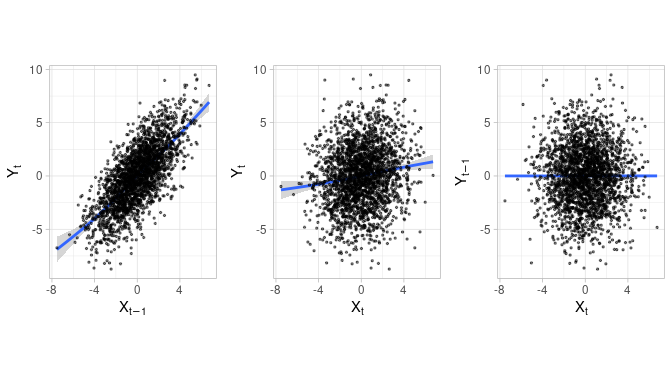We estimate Shannon transfer entropy with the defaults for all function arguments and using the parallel processing option provided by the future package. We provide more information about the parallel backend later in this vignette.

library(future)
# enable parallel processing for all future transfer_entropy calls
plan(multiprocess)
#> Warning: Strategy 'multiprocess' is deprecated in future (>= 1.20.0). Instead,
#> explicitly specify either 'multisession' or 'multicore'. In the current R
#> session, 'multiprocess' equals 'multisession'.
#> Warning in supportsMulticoreAndRStudio(...): [ONE-TIME WARNING] Forked
#> processing ('multicore') is not supported when running R from RStudio
#> because it is considered unstable. For more details, how to control forked
#> processing or not, and how to silence this warning in future R sessions, see ?
#> parallelly::supportsMulticore
set.seed(12345)
shannon_te <- transfer_entropy(x, y)
#> Shannon's entropy on 8 cores with 100 shuffles.
#>   x and y have length 2500 (0 NAs removed)
#>   [calculate] X->Y transfer entropy
#>   [calculate] Y->X transfer entropy
#>   [bootstrap] 300 times
#> Done - Total time 4.55 seconds

During the estimation process, RTransferEntropy provides information on the type of transfer entropy that is currently being estimated, the number of cores used for parallel computing, the number of shuffles and bootstrap estimations (for each direction) as well es the length of the time series and the number of removed NAs (if any). The total time in seconds is displyed after the estimation is done. The output of transfer_entropy() (see below) is closely modelled to the typical regression output tables in R and summarizes all important information. For each direction of the (possible) information flow, the Shannon transfer entropy is given in the TE column. Effective transfer entropy estimates for both direction can be found in the Eff.TE column. Standard errors and p-values in the fourth and fifth columns are based on the bootstrap samples, whose quantiles are depicted in the lower part of the ouput table. The TE estimates are compared to the quantiles of the bootstrap samples to calculate p-values and to provide an easy-to-read indication of statistical significance in the last column, according to the definition below the output table. From the output below, we can see that there is a significant information flow from $$X$$ to $$Y$$ but not vice versa, as expected due to the simulated processes.

shannon_te
#> Shannon Transfer Entropy Results:
#> -----------------------------------------------------------
#> Direction        TE   Eff. TE  Std.Err.   p-value    sig
#> -----------------------------------------------------------
#>      X->Y    0.0933    0.0901    0.0013    0.0000    ***
#>      Y->X    0.0025    0.0000    0.0013    0.6800
#> -----------------------------------------------------------
#> Bootstrapped TE Quantiles (300 replications):
#> -----------------------------------------------------------
#> Direction      0%     25%     50%     75%    100%
#> -----------------------------------------------------------
#>      X->Y  0.0009  0.0023  0.0030  0.0039  0.0084
#>      Y->X  0.0008  0.0024  0.0030  0.0039  0.0106
#> -----------------------------------------------------------
#> Number of Observations: 2500
#> -----------------------------------------------------------
#> p-values: < 0.001 '***', < 0.01 '**', < 0.05 '*', < 0.1 '.'

If we only want to calculate the transfer entropy from $$X$$ to $$Y$$ (for the opposite flow we would simple have to reverse the input parameters) and omit the bootstrap and other calculations, we can use the calc_te function (or the calc_ete function for the effective transfer entropy).

# X->Y
calc_te(x, y)
#>  0.09331606
calc_ete(x, y)
#>  0.09022173

# and Y->X
calc_te(y, x)
#>  0.002455513
calc_ete(y, x)
#>  0

Note that the effective transfer entropy relies on a random component (induced by shuffling and repeated reestimation). Therefore, the results might be slightly different.

### Simulated series II

Consider again the above example, where the results show a significant information flow from $$X$$ to $$Y$$, but not vice versa. Similar conclusions could be drawn from using a vector autoregressive model and testing for Granger causality. However, the main advantage of using transfer entropy is that it is not limited to linear relationships. Consider the following nonlinear relation between $$X$$ and $$Y$$, where, again, only $$Y$$ depends on $$X$$: $\begin{split} x_t & = & 0.2x_{t-1} + \varepsilon_{x,t}\\ y_t & = & \sqrt{\mid x_{t-1}\mid} + \varepsilon_{y,t}, \end{split}$ with $$\varepsilon_{x,t}$$ and $$\varepsilon_{y,t}$$ being standard normally distributed. We simulate these processes, discarding the first 200 observations as a burn-in period.

set.seed(12345)
n <- 2500
x <- rep(0, n + 200)
y <- rep(0, n + 200)

x <- rnorm(1, 0, 1)
y <- rnorm(1, 0, 1)

for (i in 2:(n + 200)) {
x[i] <- 0.2 * x[i - 1] + rnorm(1, 0, 1)
y[i] <- sqrt(abs(x[i - 1])) + rnorm(1, 0, 1)
}

x <- x[-(1:200)]
y <- y[-(1:200)]

As in the previous example, a scatterplot provides a first impression of the dependencies among both time series for different lead-lag combinations.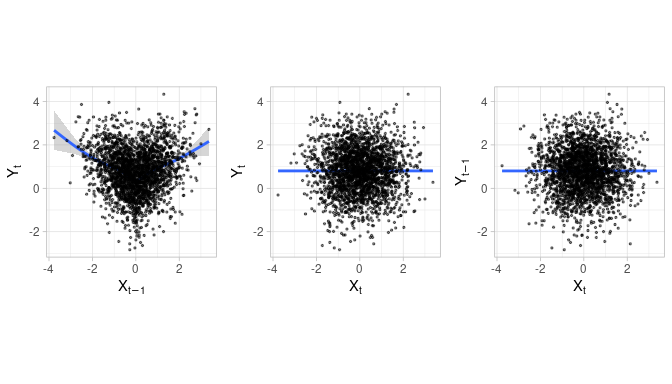The focus of this example is on Shannon transfer entropy. We use the standard settings of the transfer_entropy() function which uses one lag to calculate the transfer entropy.

shannon_te2 <- transfer_entropy(x, y)
#> Shannon's entropy on 8 cores with 100 shuffles.
#>   x and y have length 2500 (0 NAs removed)
#>   [calculate] X->Y transfer entropy
#>   [calculate] Y->X transfer entropy
#>   [bootstrap] 300 times
#> Done - Total time 3.94 seconds

shannon_te2
#> Shannon Transfer Entropy Results:
#> -----------------------------------------------------------
#> Direction        TE   Eff. TE  Std.Err.   p-value    sig
#> -----------------------------------------------------------
#>      X->Y    0.0147    0.0111    0.0012    0.0000    ***
#>      Y->X    0.0032    0.0000    0.0011    0.5233
#> -----------------------------------------------------------
#> Bootstrapped TE Quantiles (300 replications):
#> -----------------------------------------------------------
#> Direction      0%     25%     50%     75%    100%
#> -----------------------------------------------------------
#>      X->Y  0.0011  0.0026  0.0033  0.0041  0.0069
#>      Y->X  0.0011  0.0023  0.0030  0.0038  0.0076
#> -----------------------------------------------------------
#> Number of Observations: 2500
#> -----------------------------------------------------------
#> p-values: < 0.001 '***', < 0.01 '**', < 0.05 '*', < 0.1 '.'

The resulting Shannon transfer entropy estimate indicates that there is a significant information flow from $$X$$ to $$Y$$, but not in the other direction.

In the same situation, using a VAR would not reveal any relationship between $$X$$ and $$Y$$. Using the package vars and one lag (the true lag structure) delivers the following estimates of the VAR(1) for the dependent variable $$Y$$:

library(vars)
varfit <- VAR(cbind(x, y), p = 1, type = "const")
svf <- summary(varfit)

svf$varresult$y
#>
#> Call:
#> lm(formula = y ~ -1 + ., data = datamat)
#>
#> Residuals:
#>     Min      1Q  Median      3Q     Max
#> -3.6282 -0.6811  0.0170  0.6941  3.5409
#>
#> Coefficients:
#>        Estimate Std. Error t value Pr(>|t|)
#> x.l1  -0.004120   0.020498  -0.201    0.841
#> y.l1   0.006781   0.020016   0.339    0.735
#> const  0.795104   0.026262  30.276   <2e-16 ***
#> ---
#> Signif. codes:  0 '***' 0.001 '**' 0.01 '*' 0.05 '.' 0.1 ' ' 1
#>
#> Residual standard error: 1.041 on 2496 degrees of freedom
#> Multiple R-squared:  6.218e-05,  Adjusted R-squared:  -0.000739
#> F-statistic: 0.07761 on 2 and 2496 DF,  p-value: 0.9253

The VAR cannot detect the nonlinear dependence of $$Y$$ on $$X$$, which leads to a parameter estimate x.l1 that is not statistically significant. The autoregressive nature of $$X$$ (not shown above, but can be obtained with svf$varresult$x) is, as expected, readily identified.

The precise value of the transfer entropy is influenced by the choice of the quantiles. To illustrate the effect, we reestimate Shannon transfer entropy for a selection of quantiles. The following graph reports the results for increasing tail bins (and, thus, a shrinking central bin).

df <- data.frame(q1 = 5:25, q2 = 95:75)

df$ete <- apply( df, 1, function(el) calc_ete(x, y, quantiles = c(el[["q1"]], el[["q2"]])) ) df$quantiles <- factor(sprintf("(%02.f, %02.f)", df$q1, df$q2))

ggplot(df, aes(x = quantiles, y = ete)) +
geom_point() +
theme(axis.text.x = element_text(angle = 90)) +
labs(x = "Quantiles", y = "ETE (X->Y)")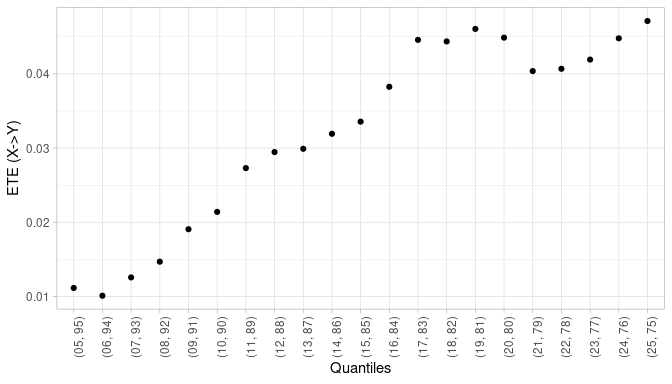As can be seen, the relative value of the effective transfer entropy from $$X$$ to $$Y$$ increases as the central bin gets smaller. Statistical significance (not shown in the plot), is, however, not affected.

### Simulated series III

As shown above, the question how to determine the quantiles is important as it impacts the order of magnitude of the transfer entropy. A different approach is provided by Rényi transfer entropy, which allows to reweight the probabilities associated with the individual bins. Due to the proposed symbolic encoding of the data, tail events are associated with a lower likelihood compared to events in the centre of the distribution. Rényi transfer entropy, thus, puts more weight on the tails when calculating transfer entropy. This is particularly convenient when the distribution is assumed to be more informative in the tails. Note again that Rényi transfer entropy estimates can potentially turn out negative.

To illustrate the use of Rényi transfer entropy, we simulate data for which the dependence of $$Y$$ on $$X$$ changes with the level of the innovation.

$\begin{split} x_t & = & 0.2x_{t-1} + \varepsilon_{x,t}\\ y_t & = & \begin{cases} \phantom{0.3}x_{t-1} + \varepsilon_{y,t} \quad \text{if } |\varepsilon_{y,t}| > s \\ 0.2x_{t-1} + \varepsilon_{y,t} \quad \text{if } |\varepsilon_{y,t}| < s \end{cases}, \end{split}$

with $$\varepsilon_{x,t}$$ and $$\varepsilon_{y,t}$$ being standard normally distributed and $$s = 2\sigma_y$$ and $$\sigma_y$$ the standard deviation of $$\varepsilon_{y,t}$$. As before, $$X$$ serves as a predictor for $$Y$$, but not vice versa.

set.seed(12345)

x <- rep(0, n + 200)
y <- rep(0, n + 200)

x <- rnorm(1, 0, 1)
y <- rnorm(1, 0, 1)

for (i in 2:(n + 200)) {
x[i] <- 0.2 * x[i - 1] + rnorm(1, 0, 1)
y[i] <- ifelse(
abs(x[i - 1]) > 1.65,
x[i - 1]  + rnorm(1, 0, 1),
0.2 * x[i - 1] + rnorm(1, 0, 1)
)
}

x <- x[-(1:200)]
y <- y[-(1:200)]

Again, a scatterplot provides a first approximation of the dependencies among both time series.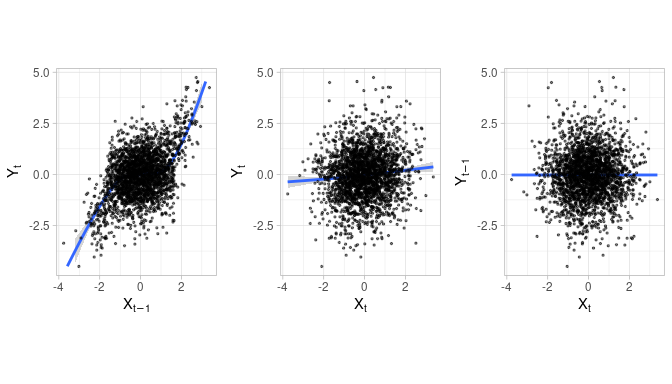In a first step, we estimate Rényi transfer entropy with a weighting parameter $$q=0.3$$, which gives a reasonable weight to the (infrequent) tail observations. Estimation of Rényi transfer entropy is, thus, invoqued as follows:

set.seed(12345)
renyi_te <- transfer_entropy(x, y, entropy = "Renyi", q = 0.3)
#> Renyi's entropy on 8 cores with 100 shuffles.
#>   x and y have length 2500 (0 NAs removed)
#>   [calculate] X->Y transfer entropy
#>   [calculate] Y->X transfer entropy
#>   [bootstrap] 300 times
#> Done - Total time 3.78 seconds

renyi_te
#> Renyi Transfer Entropy Results:
#> -----------------------------------------------------------
#> Direction        TE   Eff. TE  Std.Err.   p-value    sig
#> -----------------------------------------------------------
#>      X->Y    0.1889    0.0584    0.0393    0.0167      *
#>      Y->X    0.0523   -0.0610    0.0385    0.9500
#> -----------------------------------------------------------
#> Bootstrapped TE Quantiles (300 replications):
#> -----------------------------------------------------------
#> Direction      0%     25%     50%     75%    100%
#> -----------------------------------------------------------
#>      X->Y  0.0028  0.0946  0.1203  0.1471  0.2320
#>      Y->X  0.0026  0.0842  0.1077  0.1387  0.2191
#> -----------------------------------------------------------
#> Number of Observations: 2500
#> Q: 0.3
#> -----------------------------------------------------------
#> p-values: < 0.001 '***', < 0.01 '**', < 0.05 '*', < 0.1 '.'

The results indicate first and foremost that there is (statistically significant) information flow from $$X$$ to $$Y$$. The effect in the other direction is not statistically significant.

The appealing property of the Rényi transfer entropy is the possibility to reweight the probabilities. Furthermore, as $$q \rightarrow 1$$, Rényi transfer entropy approaches Shannon transfer entropy. We illustrate these two effects using different values of $$q$$ in the estimation of Rényi transfer entropy and compare the result with the Shannon transfer entropy. Note that the values are only comparable when the same bins are used in the symbolic encoding. Furthermore, the results are illustrated using the raw transfer entropy estimates and not the effective transfer entropy estimates because the latter depend on the shuffling procedure.

qs <- c(seq(0.1, 0.9, 0.1), 0.99)

te <- sapply(qs, function(q) calc_te(x, y, entropy = "renyi", q = q))
names(te) <- sprintf("q = %.2f", qs)

te_shannon <- calc_te(x, y)
te_shannon
#>  0.1485303

The Shannon transfer entropy from $$X$$ to $$Y$$ is estimated as 0.1485. Using different values of $$q$$ we obtain the following results for the Rényi transfer entropy.

round(te, 4)
#> q = 0.10 q = 0.20 q = 0.30 q = 0.40 q = 0.50 q = 0.60 q = 0.70 q = 0.80
#>   0.2910   0.2327   0.1889   0.1594   0.1429   0.1364   0.1365   0.1402
#> q = 0.90 q = 0.99
#>   0.1447   0.1482

text_df <- data.frame(x = 0.25,
y = te_shannon,
lab = sprintf("Shannon's TE = %.4f", te_shannon))

ggplot(data.frame(x = qs, y = te), aes(x = x, y = y)) +
geom_hline(yintercept = te_shannon, color = "red", linetype = "dashed") +
geom_smooth(se = F, color = "black", size = 0.5) +
theme_light() +
labs(x = "Values for q", y = "Renyi's Transfer Entropy",
title = "Renyi's Transfer Entropy for different Values of q") +
geom_text(data = text_df,
aes(label = lab), color = "red", nudge_y = 0.01)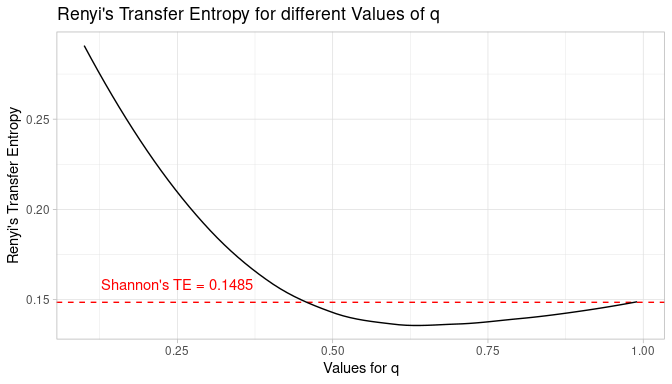As can be seen, the value of Rényi transfer entropy is highest for small values of $$q$$ and decreases as $$q$$ increases. Furthermore, it is indeed approaching 0.1485 as $$q\rightarrow 1$$.

## Application to financial time series

The analysis of information flows in financial markets has a long history. Using transfer entropy widens the possibilities to detect information flows as nonlinear relationships can also be accounted for. To illustrate the application of transfer entropy, we use a dataset of 10 individual stocks comprised in the S&P 500 index as well as the index itself. The data range from January 3, 2000, to December 29, 2017. This dataset is included as the stocks object in the package.

A stock market index is a weighted average of individual stocks. Of course, small stocks have less weight and, hence, may only have limited impact on the index while large corporations might dominate. On the other hand, it is unclear upfront how the market environment as a whole (as measured by the index) might provide information to the individual stocks. To measure the extent to which information flows between the index and the stocks, we use transfer entropy. As the calculation of transfer entropy requires stationary data, we calculate log-returns from the price series.

library(data.table) # for data manipulation

res <- lapply(split(stocks, stocks$ticker), function(d) { te <- transfer_entropy(d$ret, d$sp500, shuffles = 50, nboot = 100, quiet = T) data.table( ticker = d$ticker,
dir = c("X->Y", "Y->X"),
coef(te)[1:2, 2:3]
)
})

df <- rbindlist(res)

# order the ticker by the ete of X->Y
df[, ticker := factor(ticker,
levels = unique(df$ticker)[order(df[dir == "X->Y"]$ete)])]

# rename the variable (xy/yx)
df[, dir := factor(dir, levels = c("X->Y", "Y->X"),
labels = c("Flow towards Market",
"Flow towards Stock"))]

ggplot(df, aes(x = ticker, y = ete)) +
facet_wrap(~dir) +
geom_hline(yintercept = 0, color = "gray") +
theme(axis.text.x = element_text(angle = 90)) +
labs(x = NULL, y = "Effective Transfer Entropy") +
geom_errorbar(aes(ymin = ete - qnorm(0.95) * se,
ymax = ete + qnorm(0.95) * se),
width = 0.25, col = "blue") +
geom_point()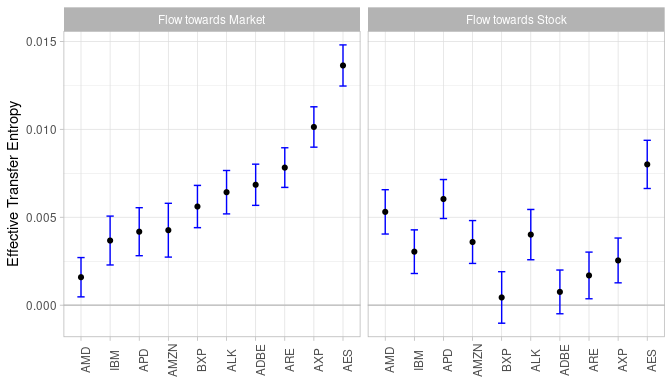The graphic reports effective transfer entropy estimates together with 95% confidence bounds for 10 stocks. The results illustrate that there is indeed bi-directional information flow. However, the information flow from the stocks to the market is higher for most stocks than the other direction. Also, as can easily be seen from the confidence bounds, the information flow towards the stock is not statistically significant in two cases (on a 5% significance level).

Again, the estimated transfer entropy depends on the choice of the quantile. This is illustrated in the following Figure which reports the changes of effective transfer entropy values for two different quantiles (05, 95) and c(10, 90) for the two flow directions: stock towards market on the left facet, and market towards stock on the right facet. The different colors represent different stocks. The estimated information flow from the market to the stocks is rather robust with respect to the choice of the quantiles, most values in both flow directions show little change (as indicated by small absolute slopes of the lines).

# calculate the same ete with different quantiles
df2 <- stocks[, .(ete_xy = calc_ete(ret, sp500, quantiles = c(10, 90)),
ete_yx = calc_ete(sp500, ret, quantiles = c(10, 90))),
by = ticker]

# combine the quantiles into a single dt

df1 <- dcast(df[, .(dir, ticker, ete)], ticker ~ dir, value.var = "ete")
setnames(df1, c("ticker", "ete_xy", "ete_yx"))
dt <- rbindlist(list(
df1[, quantiles := "(05, 95)"],
df2[, quantiles := "(10, 90)"]
))

df_long2 <- melt(dt, id.vars = c("ticker", "quantiles"))

df_long2[, quantiles := factor(quantiles, levels = c("(05, 95)", "(10, 90)"))]
df_long2[, variable := factor(variable, levels = c("ete_xy", "ete_yx"),
labels = c("Flow towards Market",
"Flow towards Stock"))]

ggplot(df_long2, aes(x = quantiles, y = value, color = ticker, group = ticker)) +
geom_line() +
facet_wrap(~variable) +
labs(
x = "Quantiles",
y = "Effective Transfer Entropy",
title = "Change of ETE-Values for different Quantiles",
color = "Ticker"
)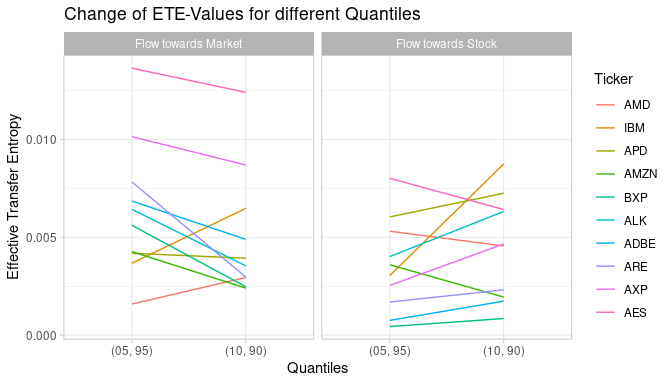In finance, pricing relevant information is readily associated with tail events, i.e. relatively large positive or negative returns. If these are indeed more relevant, Rényi transfer entropy provides a tool to give more weight to their contribution to the overall information flow. To illustrate this feature we use one stock and calculate Rényi transfer entropy from the index to the stock for a selection of the weighting parameters $$q$$.

qs <- c(seq(0.1, 0.9, 0.1), 0.99)
d <- stocks[ticker == "AXP"]

q_list <- lapply(qs, function(q) {

# transfer_entropy will give a warning as nboot < 100
suppressWarnings({
tefit <- transfer_entropy(d$ret, d$sp500, lx = 1, ly = 1,
entropy = "Renyi", q = q,
shuffles = 50, quantiles = c(10, 90),
nboot = 20, quiet = T)
})
data.table(
q   = q,
dir = c("X->Y", "Y->X"),
coef(tefit)[, 2:3]
)
})
qdt <- rbindlist(q_list)

sh_dt <- data.table(
dir = c("X->Y", "Y->X"),
ete = c(calc_ete(d$ret, d$sp500), calc_ete(d$sp500, d$ret))
)
qdt[, pe := qnorm(0.95) * se]

ggplot(qdt, aes(x = q, y = ete)) +
geom_hline(yintercept = 0, color = "darkgray") +
geom_hline(data = sh_dt, aes(yintercept = ete), linetype = "dashed",
color = "red") +
geom_point() +
geom_errorbar(aes(ymin = ete - pe, ymax = ete + pe),
width = 0.25/10, col = "blue") +
facet_wrap(~dir) +
labs(x = "Values for q", y = "Renyi's Transfer Entropy",
title = "Renyi's Transfer Entropy for different Values of q",
subtitle = "For American Express (AXP, X) and the S&P 500 Index (Y)")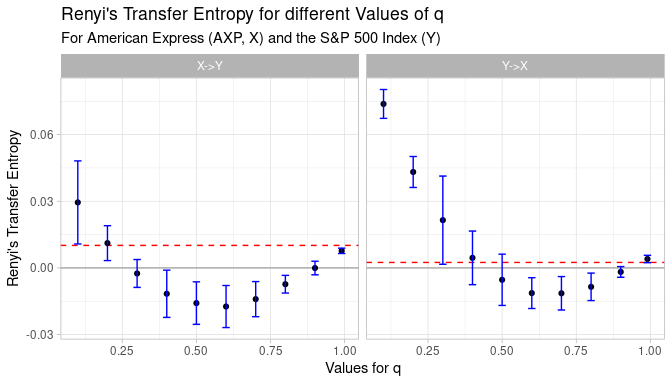For low values of $$q$$, the information in the tails is given a high weight which leads in the current situation to a significant effective transfer entropy result. This indicates that indeed tail dependence is given between the S&P 500 and the stock. As the weight is reduced, the effective transfer entropy decreases and even becomes negative (between $$q=0.3$$ and \$q=0.8 in the direction from the stock to the index and between $$q=0.5$$ and $$q=0.9$$ in the other direction). This would mean that the knowledge of the either the stock or the index would even indicate a higher risk expose of the respective other entity. Note that this does not mean that there is no information flow. The information flow merely suggests a higher risk of the dependent variable as opposed to a situation with positive Rényi transfer entropy where the risk about the dependent variable is reduced by knowledge of the other variable.

The red dashed line in the graph represents the Shannon entropy. The last value of $$q$$ is 0.99 for which the Rényi transfer entropy is already fairly close to the value of the Shannon transfer entropy. This illustrates that Rényi transfer entropy converges to Shannon transfer entropy as $$q$$ approaches 1.

## Parallel execution

RTransferEntropy uses the future package internally that allows for parallel execution. To enable parallel execution, you have to select a plan (see also ?future::plan). The following code uses multiple cores as set by plan(multiprocess) then it reverts to sequential execution.

library(future)

# enable parallelism
plan(multiprocess)
te <- transfer_entropy(x, y, nboot = 100)

# execute sequential again
plan(sequential)
te <- transfer_entropy(x, y, nboot = 100)

Turning the function to quiet = TRUE might further help to reduce time when the function is called repeatedly. If you want to disable output for all calls, you can use set_quiet(TRUE).

set_quiet(TRUE)
te <- transfer_entropy(x, y, nboot = 0)

set_quiet(FALSE)
te <- transfer_entropy(x, y, nboot = 0)
#> Shannon's entropy on 8 cores with 100 shuffles.
#>   x and y have length 2500 (0 NAs removed)
#>   [calculate] X->Y transfer entropy
#>   [calculate] Y->X transfer entropy
#> Done - Total time 0.15 seconds

## Comparison with existing package

The authors of this package are aware of one other package that calculates transfer entropy, the TransferEntropy-package. This package allows the user to compute the Shannon transfer entropy measure using the computeTE()-function and returns a single value for the calculated transfer entropy. The present package also provides the computation of Shannon transfer entropy, but is not limited to it. By default (using the transfer_entropy-function), it computes transfer entropy, effective transfer entropy (Eff. TE), standard errors, and p-values for both directions between the input time series. Standard errors are based on a bootstrap and bootstrapped transfer entropy quantiles are also provided by default. The focus of this package, therefore, is to allow the researcher to conduct inference about hypotheses regarding effective transfer entropies.

Furthermore, the present package also allows the calculation of Rényi transfer entropy. The latter is particularly useful if the tails are assumed to be more informative than the centre of the distribution.

The important difference between the TransferEntropy-package and this package is the way the data are discretized. The former package relies on the k-nearest neighbor approach of Kraskov to estimate mutual information. This package uses symbolic encoding based on selected bins or quantiles of the empirical distribution of the data to estimate empirical frequencies.

As in particular the bootstrap is computationally intensive, this package uses Rcpp and parallel processing to decrease computing time.